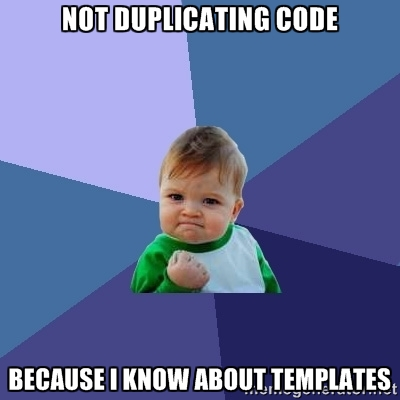C++的多态性比Objective-C的多态性更进一层。因此，当我解释这个强大的功能时要紧跟我的思路。

``````class Foo {
public:
int value() { return 5; }
};

class Bar : public Foo {
public:
int value() { return 10; }
};
``````

``````Bar *b = new Bar();
Foo *f = (Foo*)b;
printf(“%i”, f->value());
// Output = 5
``````

``````class Foo {
public:
virtual int value() { return 5; }
};

class Bar : public Foo {
public:
virtual int value() { return 10; }
};
``````

``````Bar *b = new Bar();
Foo *f = (Foo*)b;
printf(“%i”, f->value());
// Output = 10
````````````MyClass a;
a.foo();
``````

``````class Foo {
public:
virtual int value() { return 5; }
};

class Bar : public Foo {
public:
virtual int value() { return 10; }
};

Bar *b = new Bar();
Foo *f = (Foo*)b;
printf(“%i”, f->value());
// Output = 10
``````

C++中定义构造函数时与类同名。正如在Objective-C中有多个初始化方法，你也可以定义多个构造函数。

``````classFoo {
private:
intx;

public:
Foo() {
x = 0;
}

Foo(intx) {
this->x = x;
}
};
``````

``````classFoo {
private:
intx;

public:
Foo() : x(0) {
}

Foo(intx) : x(x) {
}
};
``````

``````classFoo {
private:
intx;

public:
Foo() : x(0) {
}

Foo(intx) : x(x) {
}
};

classBar :publicFoo {
private:
inty;

public:
Bar() : Foo(), y(0) {
}

Bar(intx) : Foo(x), y(0) {
}

Bar(intx,inty) : Foo(x), y(y) {
}
};
``````

C++没有一个指定的初始化程序。目前，没有办法调用同一个类的构造函数。在Objective-C中，有一个指定的初始化程序可以被其他初始化程序调用，并且只有这个指定的初始化程序去调用超类的指定初始化程序，例如：

``````@interface Foo : NSObject
@end

@implementation Foo

- (id)init {
if (self = [super init]) { ///< Call to super’s designated initialiser
}
return self;
}

- (id)initWithFoo:(id)foo {
if (self = [self init]) { ///< Call to self’s designated initialiser
// …
}
return self;
}

- (id)initWithBar:(id)bar {
if (self = [self init]) { ///< Call to self’s designated initialiser
// …
}
return self;
}

@end
``````

``````classBar :publicFoo {
private:
inty;
voidcommonInit() {
// Perform common initialisation
}

public:
Bar() : Foo() {
this->commonInit();
}

Bar(inty) : Foo(), y(y) {
this->commonInit();
}
};
``````

 然而，这十分麻烦。为什么你不能用Bar(int y)调用Bar(),然后在Bar()中这样写“Bar::commonInit()”呢？毕竟，Objective-C中恰恰是这样写的。

2011年发布了最新版的C++标准：C++11。在这个更新的标准中确实可以这样做。目前仍有许多C++代码还没有按C++11标准来更新，所以知道这两种方法很重要。任何2011年前标准的C++代码都按以下这种方式：

``````classBar :publicFoo {
private:
inty;

public:
Bar() : Foo() {
// Perform common initialisation
}

Bar(inty) : Bar() {
this->y = y;
}
};
``````

``````class Foo {
public:
~Foo() {
printf(“Foo destructor\n”);
}
};
``````

``````class Bar : public Foo {
public:
~Bar() {
printf(“Bar destructor\n”);
}
};
``````

``````Bar *b = new Bar();
Foo *f = (Foo*)b;
delete f;
// Output:
// Foo destructor
``````

``````classFoo {
public:
virtual~Foo() {
printf(“Foo destructor\n”);
}
};

classBar :publicFoo {
public:
virtual~Bar() {
printf(“Bar destructor\n”);
}
};

Bar *b =newBar();
Foo *f = (Foo*)b;
deletef;
// Output:
// Bar destructor
// Foo destructor
````````````intx = 5;
inty = x + 5;///< y = 10
``````

``````intx = 5;
``````

``````classDoubleInt {
private:
intx;
inty;

public:
DoubleInt(intx,inty) : x(x), y(y) {}
};
``````

``````DoubleInt a(1, 2);
DoubleInt b(3, 4);
DoubleInt c = a + b;
``````

``````DoubleInt operator+(constDoubleInt &rhs) {
returnDoubleInt(x + rhs.x, y + rhs.y);
}
``````

C++能做的不仅是这些。你可能不仅仅想把DoubleInt添加至DoubleInt。你可能想要给DoubleInt添加一个整数。这些都是可能实现的！

``````DoubleInt operator+(constint&rhs) {
returnDoubleInt(x + rhs, y + rhs);
}
``````

``````DoubleInt a(1, 2);
DoubleInt b = a + 10;
// b = DoubleInt(11, 12);
``````

``````voidswap(int&a,int&b) {
inttemp = a;
a = b;
b = temp;
}
``````

``````voidswap(float&a,float&b) {
floattemp = a;
a = b;
b = temp;
}
``````

``````template<typenameT>
voidswap(T a, T b) {
T temp = a;
a = b;
b = temp;
}
``````

``````intix = 1, iy = 2;
swap(ix, iy);

floatfx = 3.141, iy = 2.901;
swap(fx, fy);

Person px(“Matt Galloway”), py(“Ray Wenderlich”);
swap(px, py);
``````

``````classIntTriplet {
private:
inta, b, c;

public:
IntTriplet(inta,intb,intc) : a(a), b(b), c(c) {}

intgetA() {returna; }
intgetB() {returnb; }
intgetC() {returnc; }
};
``````

``````classFloatTriplet {
private:
floata, b, c;

public:
FloatTriplet(floata,floatb,floatc) : a(a), b(b), c(c) {}

floatgetA() {returna; }
floatgetB() {returnb; }
floatgetC() {returnc; }
};
``````

``````template<typenameT>
classTriplet {
private:
T a, b, c;

public:
Triplet(T a, T b, T c) : a(a), b(b), c(c) {}

T getA() {returna; }
T getB() {returnb; }
T getC() {returnc; }
};
``````

``````Triplet<int> intTriplet(1, 2, 3);
Triplet<float> floatTriplet(3.141, 2.901, 10.5);
Triplet<Person> personTriplet(Person(“Matt”), Person(“Ray”), Person(“Bob”));
````````````template<typenameTA,typenameTB,typenameTC>
classTriplet {
private:
TA a;
TB b;
TC c;

public:
Triplet(TA a, TB b, TC c) : a(a), b(b), c(c) {}

TA getA() {returna; }
TB getB() {returnb; }
TC getC() {returnc; }
};
``````

1 Triplet mixedTriplet(1, 3.141, Person(“Matt”)); 以上为模板的间接。接下来看看大量使用其特性的一个库–标准模板库

STL中有很多内容，要介绍所有需要很长时间，所以在这里我只介绍一些重要的。

``````#include <vector>

std::vector<int> v;
v.push_back(1);
v.push_back(2);
v.push_back(3);
v.push_back(4);
v.push_back(5);
``````

``````intfirst = v;
intoutOfBounds = v.at(100);
``````

vector被实现为一个单一的或连续的内存块。vector的空间大小等于所存储的对象的大小乘以vector中对象数（存储4字节或者8字节的整数取决于你使用的体系结构是32位还是64位的）。

std::list与std::vector很相似。但是，list的实现方式稍稍有些不同。不是作为一个连续的内存块被实现而是作为一个双向链表被实现。这意味着，list中每个的元素都包含一个数据，一个指向前一个元素的指针和一个指向后一个元素的指针。

``````#include <list>

std::list<int> l;
l.push_back(1);
l.push_back(2);
l.push_back(3);
l.push_back(4);
l.push_back(5);
``````

``````std::list<int>::iterator i;
for(i = l.begin(); i != l.end(); i++) {
intthisInt = *i;
// Do something with thisInt
}
``````

Shared Pointer

Shared Pointer是一个对象，这个对象定义一个指针以便在underlying pointer中实现引用计数。这与在Objective-C中在ARC下使用指针是相同的。例如，以下例子展示了如何用智能指针来定义一个指针去指向一个整数：

``````std::shared_ptr<int> p1(newint(1));
std::shared_ptr<int> p2 = p1;
std::shared_ptr<int> p3 = p1;
``````

``````std::shared_ptr<int> p1(newint(1));///< Use count = 1

if(doSomething) {
std::shared_ptr<int> p2 = p1;///< Use count = 2;
// Do something with p2
}

// p2 has gone out of scope and destroyed, so use count = 1

p1.reset();

// p1 reset, so use count = 0
// The underlying int* is deleted
``````

``````std::shared_ptr<Person> p1(newPerson(“Matt Galloway”));

Person *underlyingPointer = *p1;///< Grab the underlying pointer

``````

Shared Pointer很好地给C++引入了引用计数的技术。当然，shared pointer也添加了一些少量的开销，但是这个开销带来了很明显的好处，所以也是值得的。

Objective-C++

C++很好，但是跟Objective-C有什么关系呢？

``````// Forward declare so that everything works below
@class ObjcClass;
class CppClass;

// C++ class with an Objective-C member variable
class CppClass {
public:
ObjcClass *objcClass;
};

// Objective-C class with a C++ object as a property
@interface ObjcClass : NSObject
@property (nonatomic, assign) std::shared_ptr<CppClass> cppClass;
@end

@implementation ObjcClass
@end

// Using the two classes above
std::shared_ptr<CppClass> cppClass(new CppClass());
ObjcClass *objcClass = [[ObjcClass alloc] init];

cppClass->objcClass = objcClass;
objcClass.cppClass = cppClass;
``````

assign的内存管理仍然是正确的，因为你使用了shared pointer。你可以使用raw pointer，但是你需要自己写一个setter来删除原来的实例并根据情况设置一个新的值。

``````// MyClass.h
#import <Foundation/Foundation.h>
#include <list>

@interface MyClass : NSObject

@property (nonatomic, assign) std::list<int> listOfIntegers;

@end

// MyClass.mm
#import “MyClass.h”

@implementation MyClass
// …
@end
``````

MyClass类的实现文件必须是.mm文件，因为它是使用C++编写的。这没有错，但是想一想如果你想要使用MyCLass类的话会发生什么呢。你需要import MyClass.h，但是这样做你引入了一个使用C++编写的文件。所以即使其他的文件不需要用C++编写，也需要使用Objective-C++来进行编译。

C++是一个伟大的语言。它与Objective-C有相似的根源，但是它选择一种很不同的方式去编写程序。总之，学习C++可以很好的理解面向对象程序。而且C++能帮助你在objective – c代码做出更好的设计决策。我鼓励你去学习更多的C++知识并自己写程序。你可以在learncpp.com中找到很多好的资源。如果你有任何评论或者疑问或者C++问题，请留言。